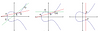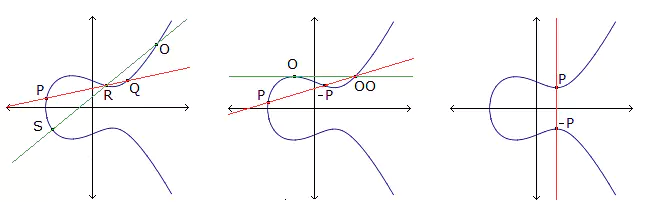# Complete $$(k,3)$$(k,3)-arcs from quartic curves

### Journal article

D. Bartoli, M. Giulietti, Giovanni Zini
Des. Codes Cryptogr., 2015

Semantic Scholar DBLP DOI
Cite

### Cite

APA
Bartoli, D., Giulietti, M., & Zini, G. (2015). Complete $$(k,3)$$(k,3)-arcs from quartic curves. Des. Codes Cryptogr.

Chicago/Turabian
Bartoli, D., M. Giulietti, and Giovanni Zini. “Complete $$(k,3)$$(k,3)-Arcs from Quartic Curves.” Des. Codes Cryptogr. (2015).

MLA
Bartoli, D., et al. “Complete $$(k,3)$$(k,3)-Arcs from Quartic Curves.” Des. Codes Cryptogr., 2015.

## Abstract

Complete $$(k,3)$$(k,3)-arcs in projective planes over finite fields are the geometric counterpart of linear non-extendible Near MDS codes of length $$k$$k and dimension $$3$$3. A class of infinite families of complete $$(k,3)$$(k,3)-arcs in $${\mathrm {PG}}(2,q)$$PG(2,q) is constructed, for $$q$$q a power of an odd prime $$p\equiv 2 ( { \, \mathrm{mod}\,}3)$$p≡2(mod3). The order of magnitude of $$k$$k is smaller than $$q$$q. This property significantly distinguishes the complete $$(k,3)$$(k,3)-arcs of this paper from the previously known infinite families, whose size differs from $$q$$q by at most $$2\sqrt{q}$$2q.

Share